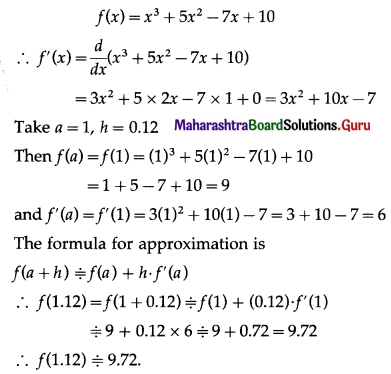Balbharti 12th Maharashtra State Board Maths Solutions Book Pdf Chapter 2 Applications of Derivatives Ex 2.2 Questions and Answers.

## Maharashtra State Board 12th Maths Solutions Chapter 2 Applications of Derivatives Ex 2.2

Question 1.
Find the approximate value of given functions, at required points.
(i) √8.95
Solution: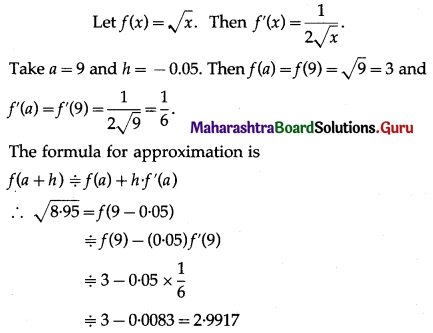√8.95 = 2.9917

(ii) $$\sqrt{28}$$
Solution: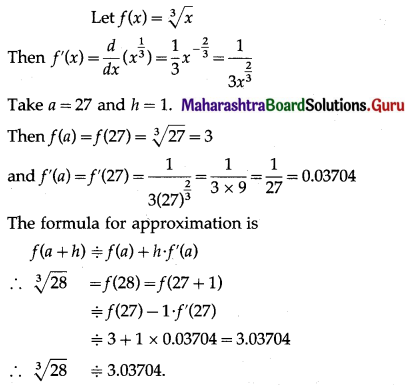(iii) $$\sqrt{31.98}$$
Solution: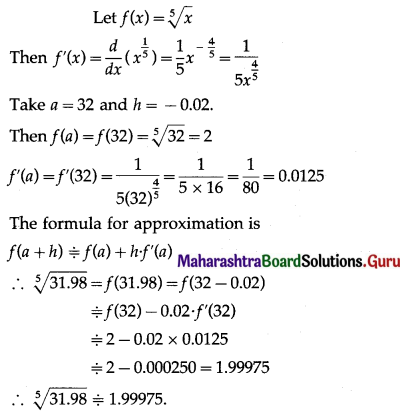(iv) (3.97)4
Solution: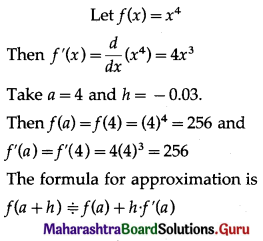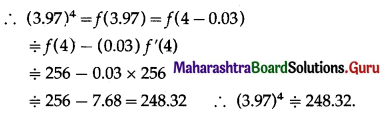(v) (4.01)3
Solution: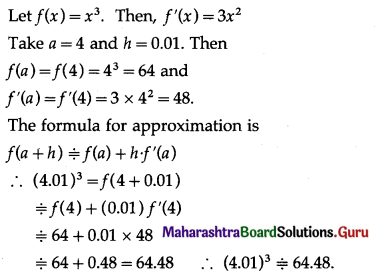Question 2.
Find the approximate values of:
(i) sin 61°, given that 1° = 0.0174c, √3 = 1.732.
Solution: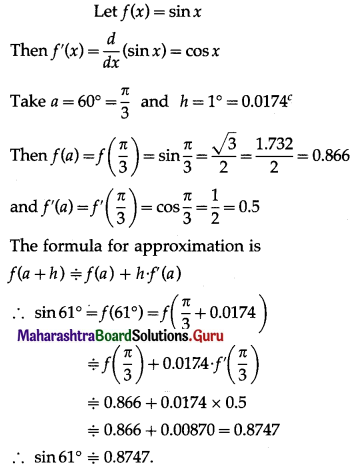(ii) sin(29° 30′), given that 1° = 0.0175c, √3 = 1.732.
Solution: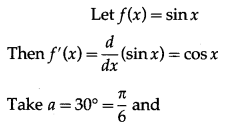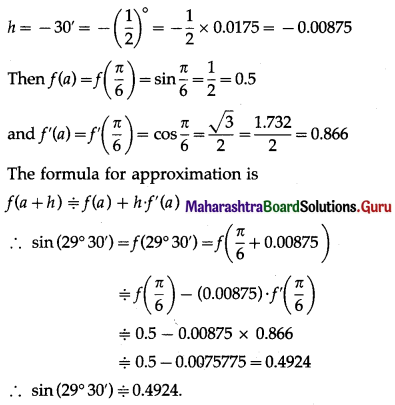(iii) cos(60° 30′), given that 1° = 0.0175c, √3 = 1.732.
Solution: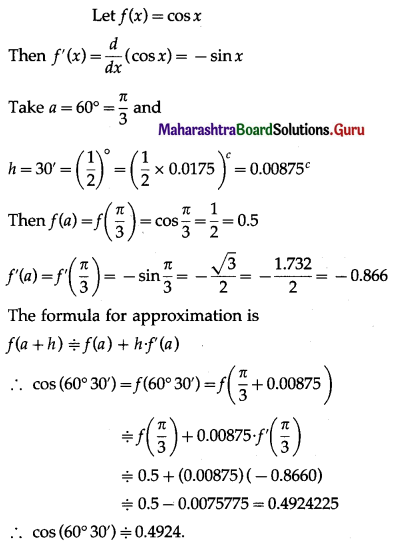(iv) tan (45° 40′), given that 1° = 0.0175c.
Solution: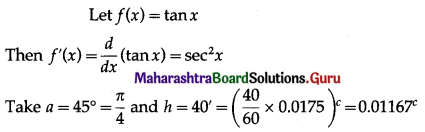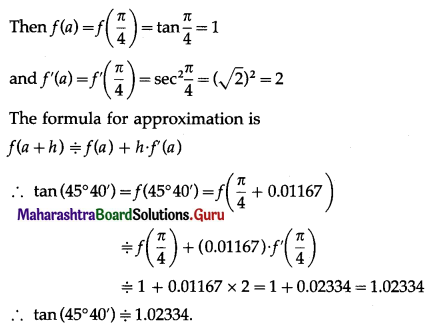Question 3.
Find the approximate values of
(i) tan-1(0.999).
Solution: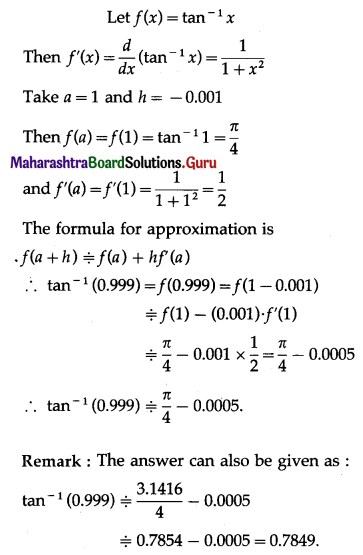(ii) cot-1(0.999).
Solution: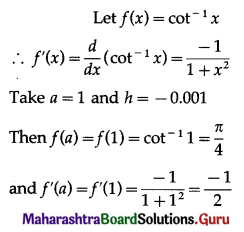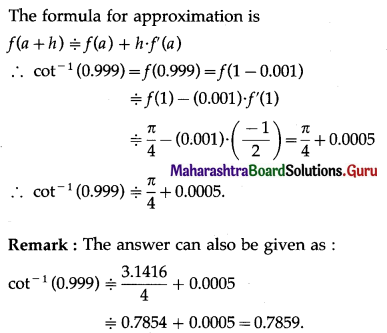(iii) tan-1(1.001).
Solution: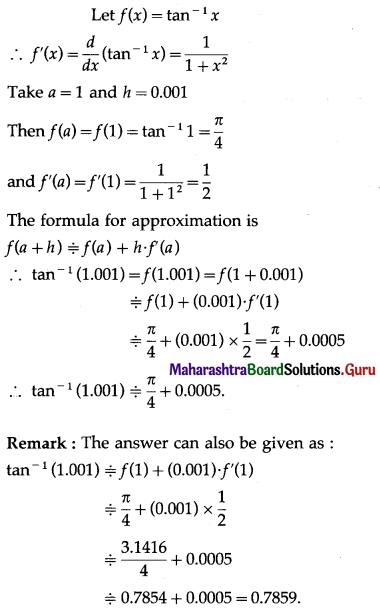Question 4.
Find the approximate values of:
(i) e0.995, given that e = 2.7183.
Solution: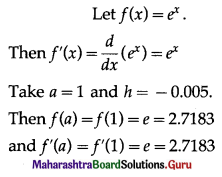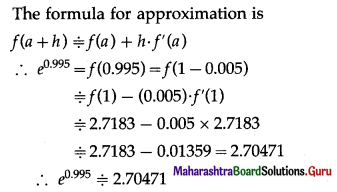(ii) e2.1, given that e2 = 7.389.
Solution: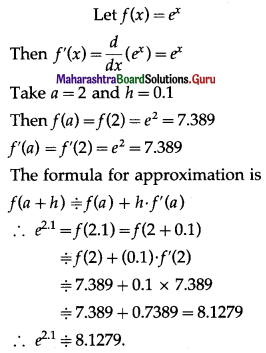(iii) 32.01, given that log 3 = 1.0986.
Solution: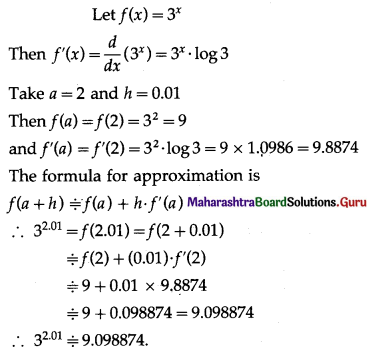Question 5.
Find the approximate values of:
(i) loge(101), given that loge10 = 2.3026.
Solution: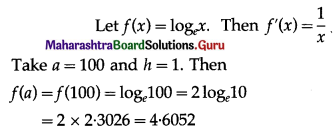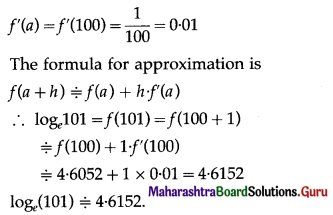(ii) loge(9.01), given that log 3 = 1.0986.
Solution: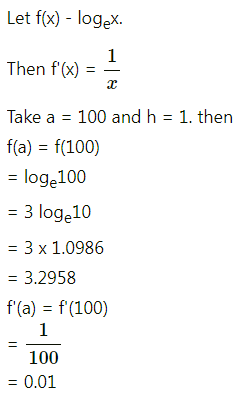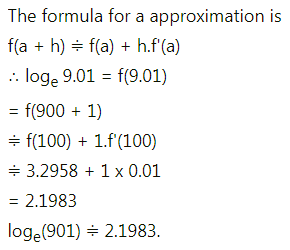(iii) log10(1016), given that log10e = 0.4343.
Solution: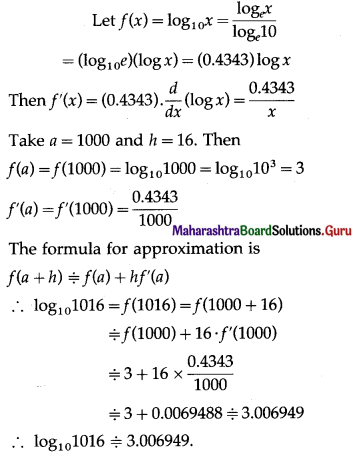Question 6.
Find the approximate values of:
(i) f(x) = x3 – 3x + 5 at x = 1.99
Solution: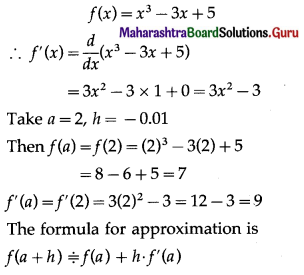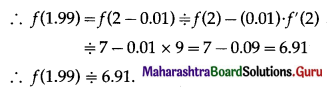(ii) f(x) = x3 + 5x2 – 7x +10 at x = 1.12
Solution: